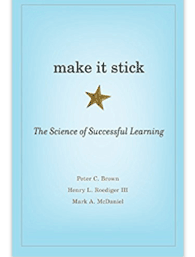A Non-profit Organization to Help Students Study, Explore, and EngageMath, Alberta Curriculum

(Free Resources)

Shortcut to Topics

In the News

Alberta challenges Grade 6 students to do math without calculators

Bridging the gender gap: why do so few girls study Stem subjects?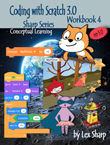Nelson, Online Resources:

Nelson Practice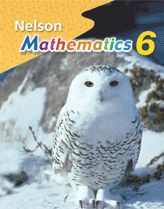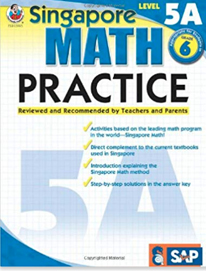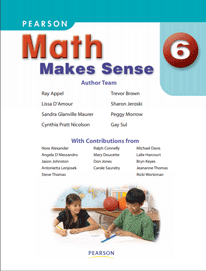Specific Topics

Shortcuts

(in alphabetical order)

Fractions
Geometry
Order of Operations
Patterns, Identifying
Place Value Unit
Percents and Rations
Probability
Rational Numbers

General Resources

Other

Assessments Questions:

Everyday Mathematics at Home - University of Chicago

Worksheets

http://www.softschools.com/math/worksheets/
https://www.math-drills.com/
http://www.homeschoolmath.net/worksheets/
http://www.worksheetfun.com/
http://gregtangmath.com/resources

Stuck with Homework?

Submit a grade 6 homework question!

Provincial Achievement Tests (PAT)

Check with your teacher, your school may have an account with exambank for more practice tests.

Hands on Activities...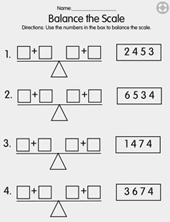Specific Topics

Place Value Unit

Place Value practice on IXL (some free practice allowed per day)

Order of Operations

Lessons

Worksheets https://www.math-drills.com/search.php?s=order+of+operations&page=1&sort=weekly

https://www.math-drills.com/orderofoperations/ooo_integers_twosteps_positive_pemdas_001.php

http://www.softschools.com/math/order_of_operations/order_of_operations_worksheets/

Identifying Patterns

Visual and Numerical Patterns

Pattern Worksheets

Numerical Patterns
Geometric Patterns
Nelson, Self-grading quizzes on patterns.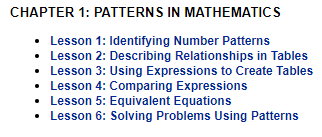Rational Numbers

Intro Intro to rational and irrational numbers

https://www.mathsisfun.com/rational-numbers.html

Write a rational number as a fraction

Worksheets Comparing rational numbers on IXL (allows a few free excercises per day)

Ordering rational numbers on IXL

Self-grading quizzes on ratinal numbers (mathopolis)

Percents and Rations

Percent lesson (interactive) http://www.learnalberta.ca/content/mesg/html/math6web/index.html?page=lessons&lesson=m6lessonshell04.swf
Rates and ratios

https://www.mathplanet.com/education/pre-algebra/ratios-and-percent/rates-and-ratios

Worksheets

Percents worksheets http://www.cimt.org.uk/projects/mepres/allgcse/bkb11.pdf

Convert between percents, fractions and decimals (IXL)

Write fraction as percent

Fractions

Activity: use mathlearningcenter.org to experiment with fractions (see the video tutorial on how to use this tool before you begin).
Fraction Resources https://bstockus.wordpress.com/fraction-resources/

Lessons: https://im.openupresources.org/6/students/index.html#

Equivalent Fractions and Proportions

https://www.mathantics.com/section/lesson-video/proportions

Equivalent Fractions Video (4 min)

Worksheets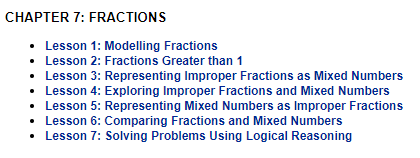Graphic fractions worksheets

https://www.math-drills.com/fractions/fractions_equivalent_visual_models_simplified_both_001.php

Operations on fractions Select the category and click generate worksheet:
http://www.softschools.com/math/worksheets/fractions.jsp

Simplifying fractions worksheets https://www.math-drills.com/fractions/simplify_fractions_hard_001.php

Geometry

Reflection http://www.learnalberta.ca/content/memg/Division02/Reflection%20(Flip)/index.html
Rotation http://www.learnalberta.ca/content/memg/Division02/Rotation%20(Turn)/index.html

Circle Geometry Part I (3 min),
Basic Concepts:
- diameter,
- sector,
- arc,
- circumference,
- parameter,

Area of Squares and Rectangles

Area of Triangles
(Math Circles Presentations,
University of Waterloo)

PDF file, solutions
(index page)
Geometry: Congruence, Constructions, and Parallel Lines http://everydaymath.uchicago.edu/parents/6th-grade/em-at-home/unit_5/

Probability

IXL topics

- a number of exercises per day are allowed free,
- search the page for "probability"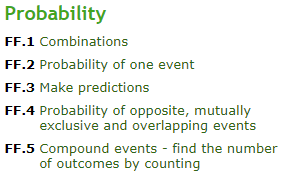Nelson, self-grading probability quizzes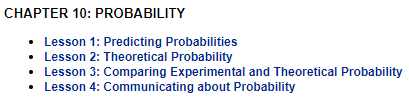Virtual Dice and Coin Flip applications https://freeonlinedice.com/
Chance experiments with spinners teacher.desmos.com

from Michigan State University:

- Factors, multiples, divisors, and products. How the Distributive Property relates multiplication and addition. (continue here to PDF).

- Fractions, decimals, ratios and percents to measure and to compare quantities (continue here to PDF).

- The four basic arithmetic operations with fractions, including mixed numbers and strategies (continue here to PDF).

- Areas and perimeters of figures and volume of rectangular prisms (continue here to PDF).

- The four operations (+, -, x, ÷,) on decimal numbers and working with percents (continue here to PDF).

- Variables and Patterns (continue here to PDF).

- Ways to collect, organize, display, and analyze data (continue here to PDF).

Related Books...

 Work on Logic Read the chapter on Venn Diagrams, which are useful in prime factorization algorithms.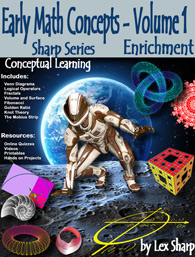Make It Stick offers techniques for becoming more productive learners, and cautions against study habits and practice routines that turn out to be counterproductive